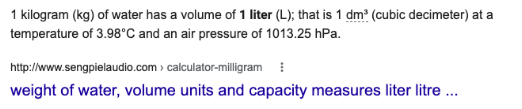## Wednesday, November 30, 2022

### Buoyancy: Notes and Such

Physics Index

Where are we going with this? The information on this page connects to standards that create understanding of the forces and interactions between objects is important for describing an object’s motion and determining the stability in a system. Students should understand that forces between objects arise from four types of interactions (gravitational, electromagnetism, and strong and weak nuclear interactions) and that some physical systems are more stable than others.

Buoyancy: Notes and Such
(Okay, this should be interesting!)

Recalling that the formula for buoyancy is

Fb  = -ρgV

a little discussion is worthwhile. First off, buoyancy is in the opposite direction of gravity. Hence, the negative sign at the front of the equation.

The rho (ρ) stands for density of the fluid. The g is acceleration due to gravity. The V is the volume of the fluid.

So, in essence, the upward force is equal to the weight of the fluid displaced.

If you displace 10 pounds of water (say you are floating a fireproof box), the upward force is 10 pounds. If you diplace 10 pounds of lava, the upward force is 10 pounds. Naturally, 10 pounds of water has a much higher volume than 10 pounds of lava.

Generally, in physics, we work with kilograms, liters, and Newtons.

When a solid object is placed in/on a fluid, it will either sink or float. If the weight of the fluid displaced before it goes under is greater than the object's weight, it will float. If not, it will sink. So, we can say the force of weight (mg) of a floating object is equal to weight of the displaced fluid on which it is floating.

Fweight = Fbuoyancy
mg = -ρgV

Conveniently, both the fluid and the object are affected by the same gravity, so the above relationship is true regarding mass, as well. The mass of a floating object is equal to the mass of the displaced fluid on which it is floating.

When an object sinks, it still displaces some fluid. Thus, there is an upward force of some amount, but it is less than the force of weight.

If you were to weigh a can of (not diet) soda in the air, then weigh it sunken in water, the weight would be less. It would decrease by an amount equal to the weight of the water displaced. (Plot twist, a lot of diet sodas in a can will float.)

One more thing before we summarize… When the fluid is water, we can enjoy the fact that 1 liter of water weighs nearly, almost exactly 1 kilogram.Source: https://www.sengpielaudio.com/calculator-milligram.htm

Floating Objects

Objects float because they displace fluid with a weight equal to theirs before they go below the surface of the fluid.
• The weight of the displaced fluid is equal to the weight of the floating object.
• The mass of the displaced fluid is equal to the mass of the floating object.
If the fluid is water, the volume of the water displaced is equal to the mass of the water displaced.

Sunken Objects

Objects sink because they don't displace enough fluid. So…
• The weight of the displaced fluid is less than the weight of the sunken object.
• The volume of the displaced fluid is equal to the volume of the sunken object.

The "underwater" weight of the sunken object is less than the "above-water" weight of the object. It is reduced by an amount equal to the weight of the displaced fluid.

If the fluid is water, the volume of the water displaced is equal to the mass of the water displaced.